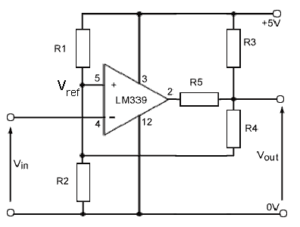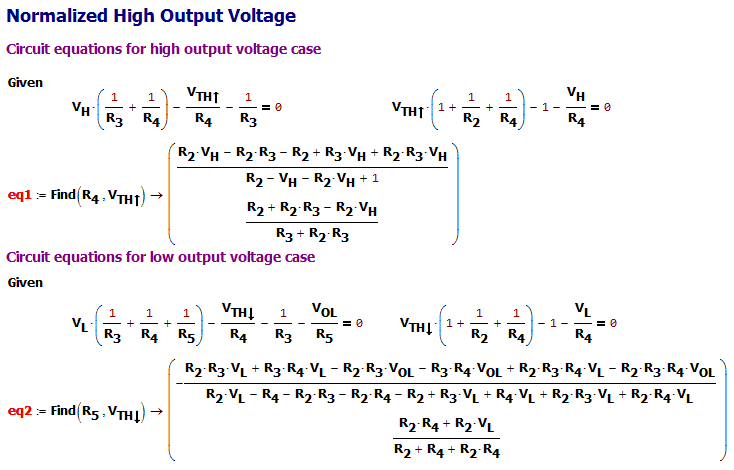# Open-Drain Comparator Circuit With Settable Trigger and Output Levels

Quote of the Day

If you don't stick to your values when they are being tested, they're not values; they're hobbies.

— Jon Stewart

## IntroductionFigure 1: An Open-Drain Schmitt Trigger Circuit with Settable Trigger and Output Levels.

I received a circuit design question from a reader who was asking how to design a comparator circuit with controllable trigger points and output levels. I have never encountered this requirement before, but it is a straightforward extension of circuits that I have covered before (example and example).

Figure 1 shows the circuit we will be working with. While simple as circuits go, you will soon see that the algebra can get quite impressive.

For those who want to work with my source, see this zip.

## Background

Let's arbitrarily selected some circuit requirements to use for an example:

• high supply voltage: VCC = 10 V
• output high voltage: VH = 8 V
• output low voltage: VL = 2 V
• rising hysteresis trigger level: $latex {{V}_{{TH\uparrow }}}=4\ \text{V}+0.4\text{ V}$
• falling hysteresis trigger level:$latex {{V}_{{TH\downarrow }}}=4\ \text{V}-0.4\text{ V}$

## Analysis

### Equation Setup

Figure 2 shows how I applied Kirchhoff's voltage law to two circuit states: output voltage high (VO=VH) and output voltage level low (VO=VL).Figure 2: Apply Kirchhoff's Voltage Law to High and Low Output Voltage Cases.

### Formulas for Resistances

Figure 3 shows how to solve for three of the resistor values (i.e. R2, R3, and R4) in terms of VH, VL, $latex {{V}_{{TH\uparrow }}}$, and $latex {{V}_{{TH\downarrow }}}$, which are set by specifications. Mathcad was not able to solve for R5 in a displayable form (i.e. too big) using these parameters, but it was able to obtain a reasonably small formula for R5 in terms of R2, R3, R4, VOL, and VL.

### Determine Component Values

Figure 4 shows how to determine the component values for this example.

### LTSpice Modeling

Figure 5 shows how I used LTSpice to capture the schematic and component values for the comparator circuit of Figure 1. The simulation results are exactly as I would have expected. For you schematic artists out there, I know I should have made a fancier symbol for the LM393 – this will have to do because I only had a couple of hours to do all this work.

## Conclusion

This is a good example of how I usually do circuit design work. I work to develop an analysis model and then move to a simulator once I have an intuitive feel for how the circuit works. I hope this answers the reader's question.

Save

Save

Save

Save

Save

Save

Save

Save

This entry was posted in Electronics. Bookmark the permalink.